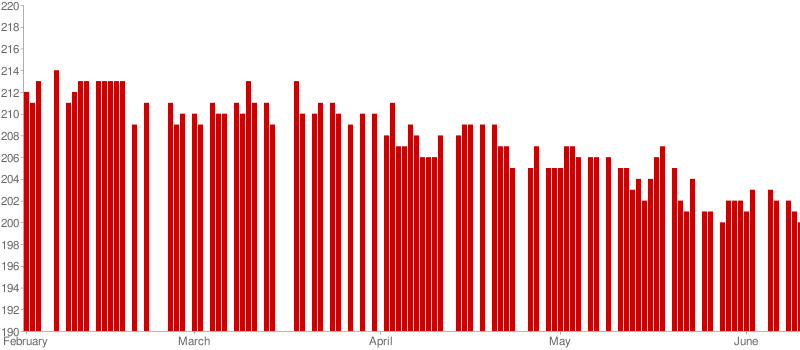# Consuming iCalendar Events with PowerShell

One of the cool benefits you get with PowerShell is the ability to make use of external .NET assemblies. Lately I found myself wanting to do some scripting involving an iCalendar feed, and since I hate writing console applications every time I have some small task to perform, PowerShell seemed like a good choice. While there is no built-in support for consuming iCalendar files, the ability to augment it with an assembly meant that I could import the DDay.iCal library I’ve used before in other .NET projects.

For this example, I had a calendar I was using in Google Calendar to track my weight. Eventually it just became a long list of numbers that would be far more interesting if they were charted. This seemed like a good chance to give the Google Chart API a spin as well.

So let’s get started. Any assemblies loaded into the .NET GAC will automatically be made available in PowerShell. For anything else, you can load it via the DLL:

[Reflection.Assembly]::LoadFile("path\\to\\DDay.iCal.dll")


And just like that, we now have full iCalendar access from our script. Now it’s time to load up the calendar and process it, sorting the events chronologically.

$calendar = [DDay.iCal.iCalendar]::LoadFromUri("http://path/to/your/calendar")$sortedEvents = $calendar.Events | Sort-Object @{Expression={$_.DTStart.Value}; Ascending=$true}  I won’t go into much detail as far as how the Google charts work so I’ll just go through my (very simplistic) implementation pretty quickly. The basic idea is that I wanted to insert blank values for any dates where I didn’t have a value, so the chart would more accurately represent the time. Since it would be messy to throw a date label on every point, I decided to just label the first of every month. Here’s the function to do that: function GetLabelFromDate($date)
{
if ($date.Day -ne 1) { return "" }$date.ToString("MMMM")
}


Next up, we’ll prepare the chart values and labels. Since the simpler options for Google Charts impose limits on y-axis values and I had no real need to do anything more complicated, I use my own axis minimum and maximum, and scale the values accordingly.

$values = @()$labels = @()
$chartMin = 190$chartMax = 220;
$valueModifier = 100 / ($chartMax - $chartMin) # process the events in the calendar foreach ($event in $sortedEvents) {$currentDate = $event.DTStart.Value$currentValue = ($event.Summary -$chartMin) * $valueModifier if ($lastDate)
{
# if there's a gap in the dates, fill it in with empty data
while ($lastDate.AddDays(1) -lt$currentDate)
{
$lastDate =$lastDate.AddDays(1)

$values += 0$labels += GetLabelFromDate $lastDate } }$labels += GetLabelFromDate $currentdate$values += $currentValue$lastDate = $currentDate } # figure out the size of the chart and bars$chartWidth = 800
$chartHeight = 350$barWidth = [Math]::Floor($chartWidth /$values.length)


Now we have the values and labels, so all we need is a chart to put them on. To do that, we need to construct the URL. Refer to the Google Charts API for documentation on what the specifics mean.

# Reference: http://code.google.com/apis/chart/docs/gallery/bar_charts.html
$url = "http://chart.apis.google.com/chart?cht=bvg&chs=$($chartWidth)x$($chartHeight)&chbh=$($barWidth),0,1&chd=t:$([String]::Join(",", $values))&chco=cc0000&chxt=x,y,r&chxl=0:$([String]::Join("|", $labels))&chxr=1,$($chartMin),$($chartMax)"  To download and display the chart, we’ll use the standard .NET WebClient class to do the heavy lifting, and download the file into my temp folder. Once it’s downloaded, the Invoke-Item cmdlet will use the default program assigned to PNG files to open it, which in my case is just the usual Windows Photo Viewer. $filename = "$env:temp\weight-chart.png"$webClient = new-object System.Net.WebClient
$Webclient.DownloadFile($url, $filename) Invoke-Item$filenameNow we have a script that will load a calendar, process the data, and chart it. Of course that’s not all the DDay.iCalendar library is capable of, or any other library you feel like importing into your script. This is just one example of how you can use existing libraries in new and interesting ways.